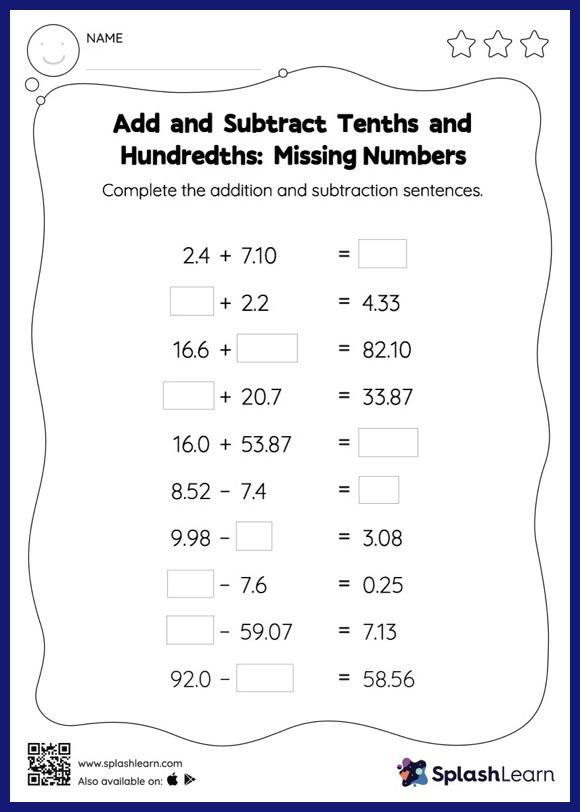# Add and Subtract Tenths and Hundredths: Missing Numbers Worksheet

Home > Add and Subtract Tenths and Hundredths: Missing NumbersThis worksheet invites students to add and subtract tenths and hundredths, which helps them become proficient in addition and subtraction. In this worksheet, students add and subtract decimals by aligning the decimal points and using zero as a placeholder. They then use the relationship between addition and subtraction to find the missing number in the add and subtract tenths and hundredths worksheet. In each problem, the numbers are laid out in the horizontal format. Students should try to use different strategies involving composing and decomposing numbers to solve these problems. This will help them develop flexibility and fluency.